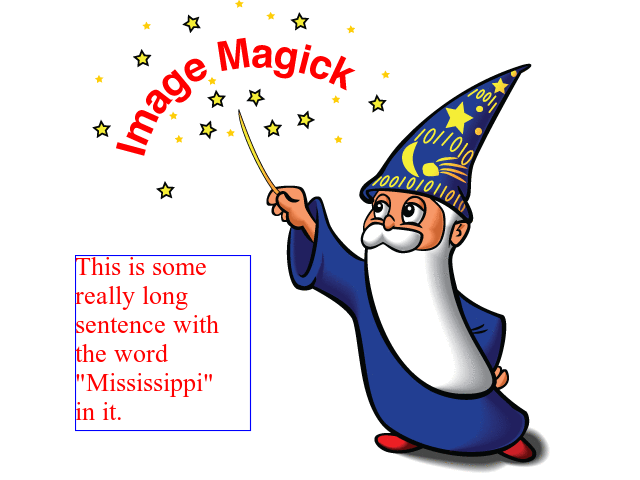# Drawing¶

New in version 0.3.0.

The `wand.drawing` module provides some basic drawing functions. `wand.drawing.Drawing` object buffers instructions for drawing shapes into images, and then it can draw these shapes into zero or more images.

It’s also callable and takes an `Image` object:

```from wand.drawing import Drawing
from wand.image import Image

with Drawing() as draw:
# does something with ``draw`` object,
# and then...
with Image(filename='wandtests/assets/beach.jpg') as image:
draw(image)
```

## Arc¶

New in version 0.4.0.

Arcs can be drawn by using `arc()` method. You’ll need to define three pairs of (x, y) coordinates. First & second pair of coordinates will be the minimum bounding rectangle, and the last pair define the starting & ending degree.

An example:

```from wand.image import Image
from wand.drawing import Drawing
from wand.color import Color

with Drawing() as draw:
draw.stroke_color = Color('black')
draw.stroke_width = 2
draw.fill_color = Color('white')
draw.arc(( 25, 25),  # Stating point
( 75, 75),  # Ending point
(135,-45))  # From bottom left around to top right
with Image(width=100,
height=100,
background=Color('lightblue')) as img:
draw.draw(img)
img.save(filename='draw-arc.gif')
```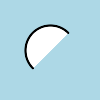## Bezier¶

New in version 0.4.0.

You can draw bezier curves using `bezier()` method. This method requires at least four points to determine a bezier curve. Given as a list of (x, y) coordinates. The first & last pair of coordinates are treated as start & end, and the second & third pair of coordinates act as controls.

For example:

```from wand.image import Image
from wand.drawing import Drawing
from wand.color import Color

with Drawing() as draw:
draw.stroke_color = Color('black')
draw.stroke_width = 2
draw.fill_color = Color('white')
points = [(10,50),  # Start point
(50,10),  # First control
(50,90),  # Second control
(90,50)]  # End point
draw.bezier(points)
with Image(width=100,
height=100,
background=Color('lightblue')) as image:
draw(image)
```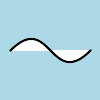Control width & color of curve with the drawing properties:

• `stroke_color`
• `stroke_width`

## Circle¶

New in version 0.4.0.

You can draw circles using `circle()` method. Circles are drawn by defining two pairs of (x, y) coordinates. First coordinate for the center “`origin`” point, and a second pair for the outer `perimeter`. For example, the following code draws a circle in the middle of the `image`:

```from wand.image import Image
from wand.drawing import Drawing
from wand.color import Color

with Drawing() as draw:
draw.stroke_color = Color('black')
draw.stroke_width = 2
draw.fill_color = Color('white')
draw.circle((50, 50), # Center point
(25, 25)) # Perimeter point
with Image(width=100, height=100, background=Color('lightblue')) as image:
draw(image)
```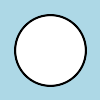## Color & Matte¶

New in version 0.4.0.

You can draw with colors directly on the coordinate system of an image. Define which color to set by setting `fill_color`. The behavior of `color()` is controlled by setting one of `PAINT_METHOD_TYPES` paint methods.

• `'point'` alters a single pixel.
• `'replace'` swaps on color for another. Threshold is influenced by `fuzz`.
• `'floodfill'` fills area of a color influenced by `fuzz`.
• `'filltoborder'` fills area of a color until border defined by `border_color`.
• `'reset'` replaces the whole image to a single color.

Example fill all to green boarder:

```from wand.drawing import Drawing
from wand.color import Color

with Drawing() as draw:
draw.border_color = Color('green')
draw.fill_color = Color('blue')
draw.color(15, 25, 'filltoborder')
```

The `matte()` method is identical to the `color()` method above, but alters the alpha channel of the color area selected. Colors can be manipulated, but not replaced.

```with Drawing() as draw:
draw.fill_color = None  # or Color('none')
draw.matte(15, 25, 'floodfill')
```

## Composite¶

New in version 0.4.0.

Similar to `composite_channel()`, this `composite()` method will render a given image on top of the drawing subject image following the `COMPOSITE_OPERATORS` options. An compositing image must be given with a destination `top`, `left`, `width`, and `height` values.

```from wand.image import Image, COMPOSITE_OPERATORS
from wand.drawing import Drawing
from wand.display import display

wizard = Image(filename='wizard:')
rose = Image(filename='rose:')

for o in COMPOSITE_OPERATORS:
w = wizard.clone()
r = rose.clone()
with Drawing() as draw:
draw.composite(operator=o, left=175, top=250,
width=r.width, height=r.height, image=r)
draw(w)
display(w)
```

## Ellipse¶

New in version 0.4.0.

Ellipse can be drawn by using the `ellipse()` method. Like drawing circles, the ellipse requires a `origin` point, however, a pair of (x, y) `radius` are used in relationship to the `origin` coordinate. By default a complete “closed” ellipse is drawn. To draw a partial ellipse, provide a pair of starting & ending degrees as the third parameter.

An example of a full ellipse:

```from wand.image import Image
from wand.drawing import Drawing
from wand.color import Color

with Drawing() as draw:
draw.stroke_color = Color('black')
draw.stroke_width = 2
draw.fill_color = Color('white')
draw.ellipse((50, 50), # Origin (center) point
(40, 20)) # 80px wide, and 40px tall
with Image(width=100, height=100, background=Color('lightblue')) as image:
draw(image)
```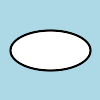Same example as above, but with a half-partial ellipse defined by the third parameter:

```draw.ellipse((50, 50), # Origin (center) point
(40, 20), # 80px wide, and 40px tall
(90,-90)) # Draw half of ellipse from bottom to top
```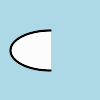## Lines¶

You can draw lines using `line()` method. It simply takes two (x, y) coordinates for start and end of a line. For example, the following code draws a diagonal line into the `image`:

```draw.line((0, 0), image.size)
draw(image)
```

Or you can turn this diagonal line upside down:

```draw.line((0, image.height), (image.width, 0))
draw(image)
```

The line color is determined by `fill_color` property, and you can change this of course. The following code draws a red diagonal line into the `image`:

```from wand.color import Color

with Color('red') as color:
draw.fill_color = color
draw.line((0, 0), image.size)
draw(image)
```

## Paths¶

New in version 0.4.0.

Paths can be drawn by using any collection of path functions between `path_start()` and `path_finish()` methods. The available path functions are:

• `path_close()` draws a path from last point to first.
• `path_curve()` draws a cubic bezier curve.
• `path_curve_to_quadratic_bezier()` draws a quadratic bezier curve.
• `path_elliptic_arc()` draws an elliptical arc.
• `path_horizontal_line()` draws a horizontal line.
• `path_line()` draws a line path.
• `path_move()` adjust current point without drawing.
• `path_vertical_line()` draws a vertical line.

Each path method expects a destination point, and will draw from the current point to the new point. The destination point will become the new current point for the next applied path method. Destination points are given in the form of (`x`, `y`) coordinates to the `to` parameter, and can by relative or absolute to the current point by setting the `relative` flag. The `path_curve()` and `path_curve_to_quadratic_bezier()` expect additional `control` points, and can complement previous drawn curves by setting a `smooth` flag. When the `smooth` flag is set to `True` the first control point is assumed to be the reflection of the last defined control point.

For example:

```from wand.image import Image
from wand.drawing import Drawing
from wand.color import Color

with Drawing() as draw:
draw.stroke_width = 2
draw.stroke_color = Color('black')
draw.fill_color = Color('white')
draw.path_start()
# Start middle-left
draw.path_move(to=(10, 50))
# Curve accross top-left to center
draw.path_curve(to=(40, 0),
controls=[(10, -40), (30,-40)],
relative=True)
# Continue curve accross bottom-right
draw.path_curve(to=(40, 0),
controls=(30, 40),
smooth=True,
relative=True)
# Line to top-right
draw.path_vertical_line(10)
# Diagonal line to bottom-left
draw.path_line(to=(10, 90))
# Close first & last points
draw.path_close()
draw.path_finish()
with Image(width=100, height=100, background=Color('lightblue')) as image:
draw(image)
```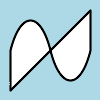## Point¶

New in version 0.4.0.

You can draw points by using `point()` method. It simply takes two `x`, `y` arguments for the point coordinate.

The following example will draw points following a math function across a given `image`:

```from wand.image import Image
from wand.drawing import Drawing
from wand.color import Color
import math

with Drawing() as draw:
for x in xrange(0, 100):
y = math.tan(x) * 4
draw.point(x, y + 50)
with Image(width=100, height=100, background=Color('lightblue')) as image:
draw(image)
```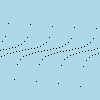Color of the point can be defined by setting the following property

• `fill_color`

## Polygon¶

New in version 0.4.0.

Complex shapes can be created with the `polygon()` method. You can draw a polygon by given this method a list of points. Stroke line will automatically close between first & last point.

For example, the following code will draw a triangle into the `image`:

```from wand.image import Image
from wand.drawing import Drawing
from wand.color import Color

with Drawing() as draw:
draw.stroke_width = 2
draw.stroke_color = Color('black')
draw.fill_color = Color('white')
points = [(25, 25), (75, 50), (25, 75)]
draw.polygon(points)
with Image(width=100, height=100, background=Color('lightblue')) as image:
draw(image)
```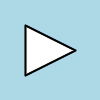Control the fill & stroke with the following properties:

• `stroke_color`
• `stroke_dash_array`
• `stroke_dash_offset`
• `stroke_line_cap`
• `stroke_line_join`
• `stroke_miter_limit`
• `stroke_opacity`
• `stroke_width`
• `fill_color`
• `fill_opacity`
• `fill_rule`

## Polyline¶

New in version 0.4.0.

Identical to `polygon()`, except `polyline()` will not close the stroke line between the first & last point.

For example, the following code will draw a two line path on the `image`:

```from wand.image import Image
from wand.drawing import Drawing
from wand.color import Color

with Drawing() as draw:
draw.stroke_width = 2
draw.stroke_color = Color('black')
draw.fill_color = Color('white')
points = [(25, 25), (75, 50), (25, 75)]
draw.polyline(points)
with Image(width=100, height=100, background=Color('lightblue')) as image:
draw(image)
```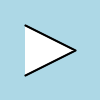Control the fill & stroke with the following properties:

• `stroke_color`
• `stroke_dash_array`
• `stroke_dash_offset`
• `stroke_line_cap`
• `stroke_line_join`
• `stroke_miter_limit`
• `stroke_opacity`
• `stroke_width`
• `fill_color`
• `fill_opacity`
• `fill_rule`

## Push & Pop¶

New in version 0.4.0.

When working with complex vector graphics, you can use ImageMagick’s internal graphic-context stack to manage different styles & operations. The methods `push()`, `push_clip_path()`, `push_defs()`, and `push_pattern()` are used to mark the beginning of a sub-routine. The clip path & pattern methods take a name based identifier argument, and can be referenced at a latter point with `clip_path`, or `set_fill_pattern_url()` / `set_stroke_pattern_url()` respectively. With stack management, `pop()` is used to mark the end of a sub-routine, and return the graphical context to its pervious state before `push()` was invoked. Methods `pop_clip_path()`, `pop_defs()`, and `pop_pattern()` exist to match there pop counterparts.

```from wand.color import Color
from wand.image import Image
from wand.drawing import Drawing
from wand.compat import nested
from math import cos, pi, sin

with nested(Color('lightblue'),
Color('transparent'),
Drawing()) as (bg, fg, draw):
draw.stroke_width = 3
draw.fill_color = fg
for degree in range(0, 360, 15):
draw.push()  # Grow stack
draw.stroke_color = Color('hsl({0}%, 100%, 50%)'.format(degree * 100 / 360))
t = degree / 180.0 * pi
x = 35 * cos(t) + 50
y = 35 * sin(t) + 50
draw.line((50, 50), (x, y))
draw.pop()  # Restore stack
with Image(width=100, height=100, background=Color('lightblue')) as img:
draw(img)
```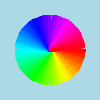## Rectangles¶

New in version 0.3.6.

Changed in version 0.4.0.

If you want to draw rectangles use `rectangle()` method. It takes `left`/`top` coordinate, and `right`/`bottom` coordinate, or `width` and `height`. For example, the following code draws a square on the `image`:

```draw.rectangle(left=10, top=10, right=40, bottom=40)
draw(image)
```

Or using `width` and `height` instead of `right` and `bottom`:

```draw.rectangle(left=10, top=10, width=30, height=30)
draw(image)
```

Support for rounded corners was added in version 0.4.0. The `radius` argument sets corner rounding.

```draw.rectangle(left=10, top=10, width=30, height=30, radius=5)
draw(image)
```

Both horizontal & vertical can be set independently with `xradius` & `yradius` respectively.

```draw.rectangle(left=10, top=10, width=30, height=30, xradius=5, yradius=3)
draw(image)
```

Note that the stoke and the fill are determined by the following properties:

• `stroke_color`
• `stroke_dash_array`
• `stroke_dash_offset`
• `stroke_line_cap`
• `stroke_line_join`
• `stroke_miter_limit`
• `stroke_opacity`
• `stroke_width`
• `fill_color`
• `fill_opacity`
• `fill_rule`

## Texts¶

`Drawing` object can write texts as well using its `text()` method. It takes `x` and `y` coordinates to be drawn and a string to write:

```draw.font = 'wandtests/assets/League_Gothic.otf'
draw.font_size = 40
draw.text(image.width / 2, image.height / 2, 'Hello, world!')
draw(image)
```

As the above code shows you can adjust several settings before writing texts:

• `font`
• `font_family`
• `font_resolution`
• `font_size`
• `font_stretch`
• `font_style`
• `font_weight`
• `gravity`
• `text_alignment`
• `text_antialias`
• `text_decoration`
• `text_direction`
• `text_interline_spacing`
• `text_interword_spacing`
• `text_kerning`
• `text_under_color`

## Word Wrapping¶

The `Drawing` class, by nature, doesn’t implement any form of word-wrapping, and users of the `wand` library would be responsible for implementing this behavior unique to their business requirements.

ImageMagick’s `caption:` coder does offer a word-wrapping solution with `Image.caption()` method, but Python’s `textwrap` is a little more sophisticated.

```from textwrap import wrap
from wand.color import Color
from wand.drawing import Drawing
from wand.image import Image

def draw_roi(contxt, roi_width, roi_height):
"""Let's draw a blue box so we can identify what
our region of intrest is."""
ctx.push()
ctx.stroke_color = Color('BLUE')
ctx.fill_color = Color('TRANSPARENT')
ctx.rectangle(left=75, top=255, width=roi_width, height=roi_height)
ctx.pop()

def word_wrap(image, ctx, text, roi_width, roi_height):
"""Break long text to multiple lines, and reduce point size
until all text fits within a bounding box."""
mutable_message = text
iteration_attempts = 100

def eval_metrics(txt):
"""Quick helper function to calculate width/height of text."""
metrics = ctx.get_font_metrics(image, txt, True)
return (metrics.text_width, metrics.text_height)

def shrink_text():
"""Reduce point-size & restore original text"""
ctx.font_size = ctx.font_size - 0.75
mutable_message = text

while ctx.font_size > 0 and iteration_attempts:
iteration_attempts -= 1
width, height = eval_metrics(mutable_message)
if height > roi_height:
shrink_text()
elif width > roi_width:
columns = len(mutable_message)
while columns > 0:
columns -= 1
mutable_message = '\n'.join(wrap(mutable_message, columns))
wrapped_width, _ = eval_metrics(mutable_message)
if wrapped_width <= roi_width:
break
if columns < 1:
shrink_text()
else:
break
if iteration_attempts < 1:
raise RuntimeError("Unable to calculate word_wrap for " + text)
return mutable_message

message = """This is some really long sentence with the
word "Mississippi" in it."""

ROI_SIDE = 175

with Image(filename='logo:') as img:
with Drawing() as ctx:
draw_roi(ctx, ROI_SIDE, ROI_SIDE)
# Set the font style
ctx.fill_color = Color('RED')
ctx.font_family = 'Times New Roman'
ctx.font_size = 32
mutable_message = word_wrap(img,
ctx,
message,
ROI_SIDE,
ROI_SIDE)
ctx.text(75, 275, mutable_message)
ctx.draw(img)
img.save(filename='draw-word-wrap.png')
```